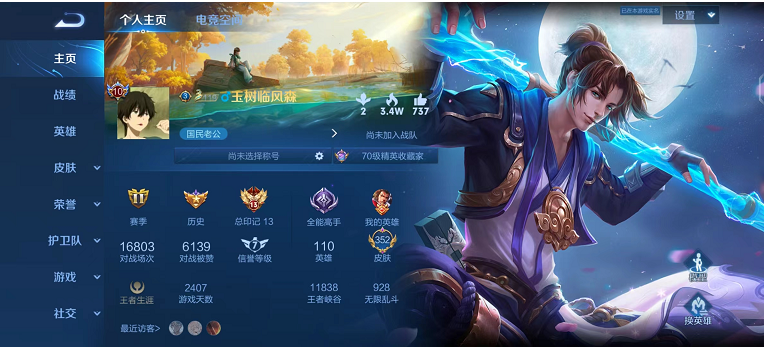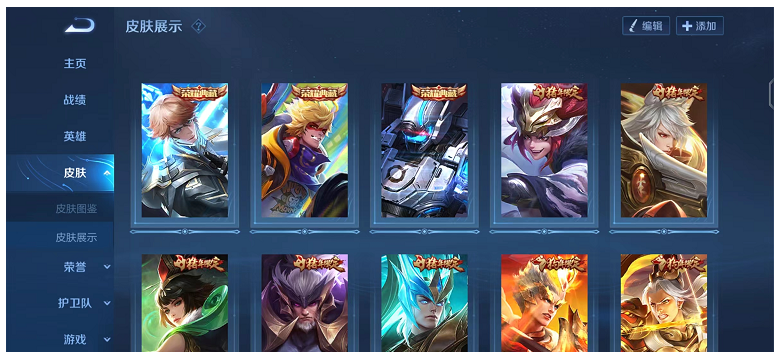AA720██ 非云██ V10星耀348皮=无限飓风号=全息碎影=无尽星芒=无双飞将=启示之音=挚爱之约=挚爱花嫁=女娲补天=花朝如约=末日机甲=淬星耀世=乞巧织情=音你心动=飞鸢探春=游龙清影

¥5.00

AA720██V10星耀348皮=无限飓风号=全息碎影=无尽星芒=无双飞将=启示之音=挚爱之约=挚爱花嫁=女娲补天=花朝如约=末日机甲=淬星耀世=乞巧织情=音你心动=飞鸢探春=游龙清影